# 1. 前言¶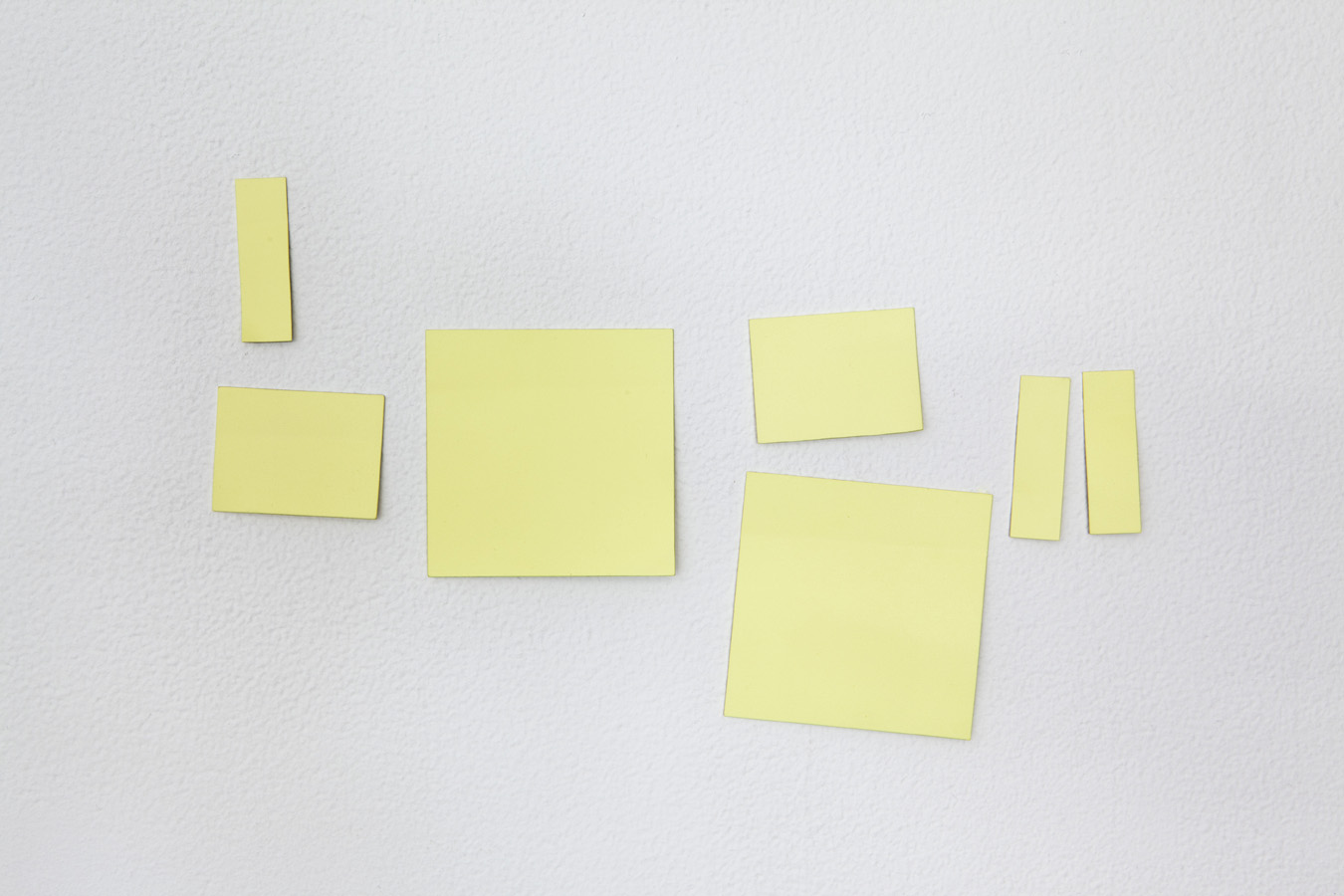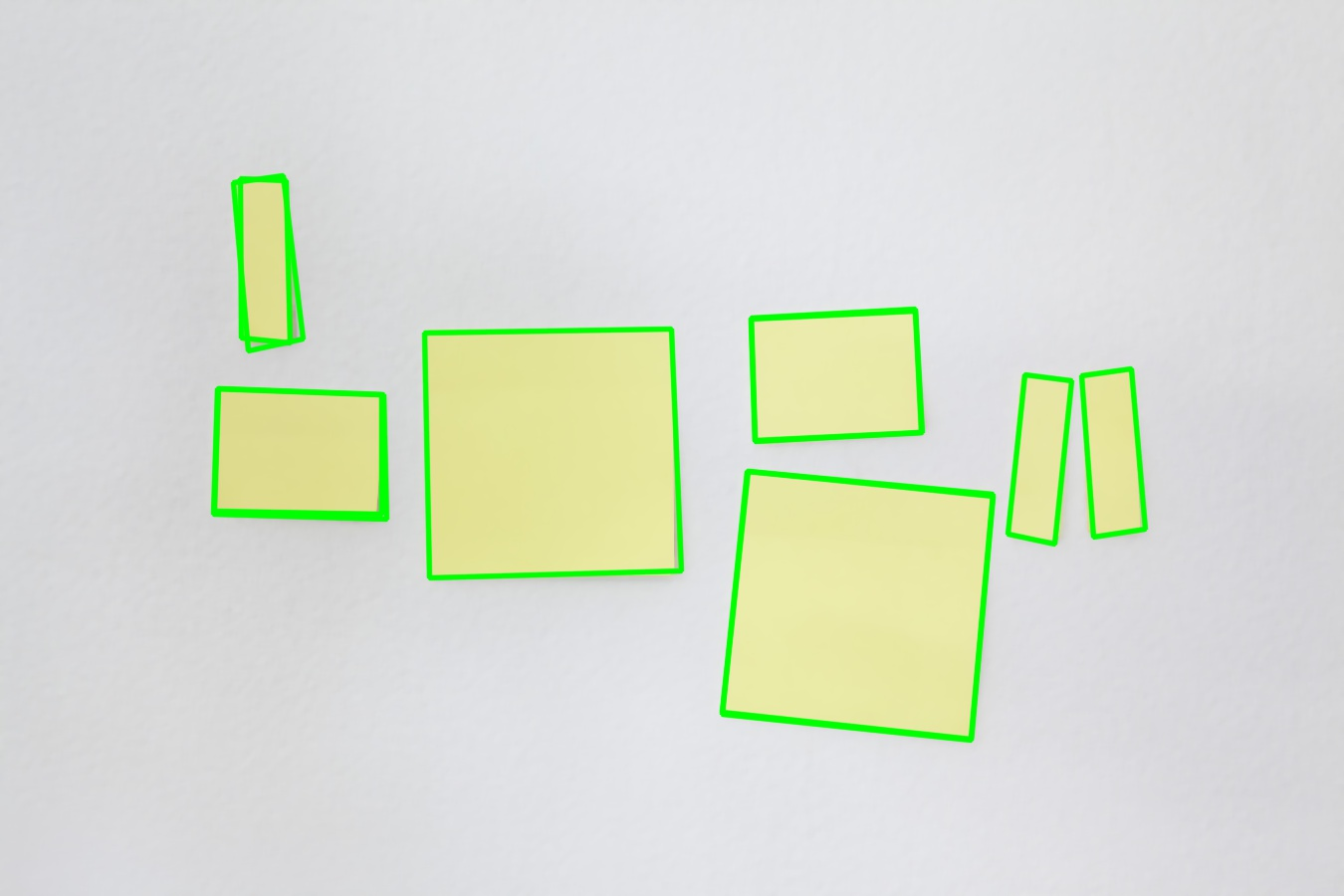# 2. 算法原理¶

• 对原始图像进行滤波。(关于滤波器的选择可以选择普通的中值滤波，也可以选择Side Window Filter的中值滤波，这取决于你是否需要图像保存更多的边缘和角点)。
• 在图像的每个颜色通道寻找矩形区域。这可以细分为：
• 在每个颜色通道对应的图像中使用不同的阈值获得对应的二值图像。
• 获得二值图像后，使用findContours算法寻找轮廓区域。
• 对于每个区域，使用approxPolyDP算法来近似轮廓为多边形。
• 对上面近似后的多边形判断顶点数是否为4，是否为凸多边形，且相邻边的夹角的cosin值是否接近0(也即是角度为90度)，如果均满足代表这个多边形为矩形，存入结果中。
• 在结果图中画出检测到的矩形区域。

# 3. 代码实现¶

const double eps = 1e-7;

//获取pt0->pt1向量和pt0->pt2向量之间的夹角
static double angle(Point pt1, Point pt2, Point pt0)
{
double dx1 = pt1.x - pt0.x;
double dy1 = pt1.y - pt0.y;
double dx2 = pt2.x - pt0.x;
double dy2 = pt2.y - pt0.y;
return (dx1*dx2 + dy1*dy2) / sqrt((dx1*dx1 + dy1*dy1)*(dx2*dx2 + dy2*dy2) + eps);
}

//寻找矩形
static void findSquares(const Mat& image, vector<vector<Point> >& squares, int N=5, int thresh=50)
{

//滤波可以提升边缘检测的性能
Mat timg(image);
// 普通中值滤波
medianBlur(image, timg, 9);
// SideWindowFilter的中值滤波
// timg = MedianSideWindowFilter(image, 4);
Mat gray0(timg.size(), CV_8U), gray;
// 存储轮廓
vector<vector<Point> > contours;

// 在图像的每一个颜色通道寻找矩形
for (int c = 0; c < 3; c++)
{
int ch[] = { c, 0 };
// 函数功能：mixChannels主要就是把输入的矩阵（或矩阵数组）的某些通道拆分复制给对应的输出矩阵（或矩阵数组）的某些通道中，其中的对应关系就由fromTo参数制定.
// 接口：void  mixChannels (const Mat*  src , int  nsrc , Mat*  dst , int  ndst , const int*  fromTo , size_t  npairs );
// src: 输入矩阵，可以为一个也可以为多个，但是矩阵必须有相同的大小和深度.
// nsrc: 输入矩阵的个数.
// dst: 输出矩阵，可以为一个也可以为多个，但是所有的矩阵必须事先分配空间（如用create），大小和深度须与输入矩阵等同.
// ndst: 输出矩阵的个数
// fromTo:设置输入矩阵的通道对应输出矩阵的通道，规则如下：首先用数字标记输入矩阵的各个通道。输入矩阵个数可能多于一个并且每个矩阵的通道可能不一样，
// 第一个输入矩阵的通道标记范围为：0 ~src.channels() - 1，第二个输入矩阵的通道标记范围为：src.channels() ~src.channels() + src.channels() - 1,
// 以此类推；其次输出矩阵也用同样的规则标记，第一个输出矩阵的通道标记范围为：0 ~dst.channels() - 1，第二个输入矩阵的通道标记范围为：dst.channels()
// ~dst.channels() + dst.channels() - 1, 以此类推；最后，数组fromTo的第一个元素即fromTo应该填入输入矩阵的某个通道标记，而fromTo的第二个元素即
// fromTo应该填入输出矩阵的某个通道标记，这样函数就会把输入矩阵的fromTo通道里面的数据复制给输出矩阵的fromTo通道。fromTo后面的元素也是这个
// 道理，总之就是一个输入矩阵的通道标记后面必须跟着个输出矩阵的通道标记.
// npairs: 即参数fromTo中的有几组输入输出通道关系，其实就是参数fromTo的数组元素个数除以2.
mixChannels(&timg, 1, &gray0, 1, ch, 1);

// 尝试几个不同的阈值
for (int l = 0; l < N; l++)
{
// hack: use Canny instead of zero threshold level.
// 在级别为0的时候不使用阈值为0，而是使用Canny边缘检测算子
if (l == 0)
{
// void Canny(  InputArray image, OutputArray edges, double threshold1, double threshold2, int apertureSize = 3, bool L2gradient = false);
// 第一个参数：输入图像（八位的图像）
// 第二个参数：输出的边缘图像
// 第三个参数：下限阈值，如果像素梯度低于下限阈值，则将像素不被认为边缘
// 第四个参数：上限阈值，如果像素梯度高于上限阈值，则将像素被认为是边缘（建议上限是下限的2倍或者3倍）
// 第五个参数：为Sobel()运算提供内核大小，默认值为3
// 第六个参数：计算图像梯度幅值的标志，默认值为false
Canny(gray0, gray, 5, thresh, 5);
// 执行形态学膨胀操作
dilate(gray, gray, Mat(), Point(-1, -1));
}
else
{
// 当l不等于0的时候，执行 tgray(x,y) = gray(x,y) < (l+1)*255/N ? 255 : 0
gray = gray0 >= (l + 1) * 255 / N;
}

// 寻找轮廓并将它们全部存储为列表
findContours(gray, contours, RETR_LIST, CHAIN_APPROX_SIMPLE);

//存储一个多边形（矩形）
vector<Point> approx;

// 测试每一个轮廓
for (size_t i = 0; i < contours.size(); i++)
{
// 近似轮廓，精度与轮廓周长成正比,主要功能是把一个连续光滑曲线折线化，对图像轮廓点进行多边形拟合。
// 函数声明：void approxPolyDP(InputArray curve, OutputArray approxCurve, double epsilon, bool closed)
// InputArray curve:一般是由图像的轮廓点组成的点集
// OutputArray approxCurve：表示输出的多边形点集
// double epsilon：主要表示输出的精度，就是两个轮廓点之间最大距离数，5,6,7，，8，，,,，
// bool closed：表示输出的多边形是否封闭

// arcLength 计算图像轮廓的周长
approxPolyDP(Mat(contours[i]), approx, arcLength(Mat(contours[i]), true)*0.02, true);

// 近似后，方形轮廓应具有4个顶点
// 相对较大的区域（以滤除嘈杂的轮廓）并且是凸集。
// 注意: 使用面积的绝对值，因为面积可以是正值或负值-根据轮廓方向
if (approx.size() == 4 &&
fabs(contourArea(Mat(approx))) > 1000 &&
isContourConvex(Mat(approx)))
{
double maxCosine = 0;

for (int j = 2; j < 5; j++)
{
// 找到相邻边之间的角度的最大余弦
double cosine = fabs(angle(approx[j % 4], approx[j - 2], approx[j - 1]));
maxCosine = MAX(maxCosine, cosine);
}

// 如果所有角度的余弦都很小(所有角度均为90度)，将顶点集合写入结果vector
if (maxCosine < 0.3)
squares.push_back(approx);
}
}
}
}
}

//在图像上画出方形
void drawSquares(Mat &image, const vector<vector<Point> >& squares) {
for (size_t i = 0; i < squares.size(); i++)
{
const Point* p = &squares[i];

int n = (int)squares[i].size();
//不检测边界
if (p->x > 3 && p->y > 3)
polylines(image, &p, &n, 1, true, Scalar(0, 255, 0), 3, LINE_AA);
}
}


# 4. 普通中值滤波的结果¶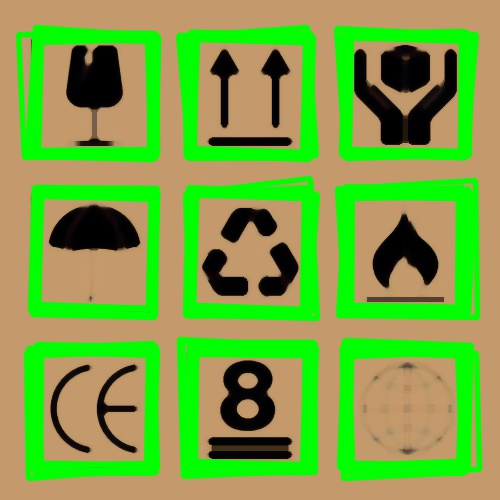# 5. 对比普通中值滤波和Side Window Filter中值滤波的结果¶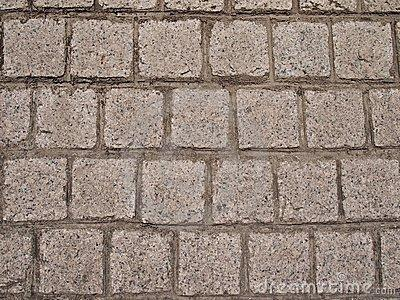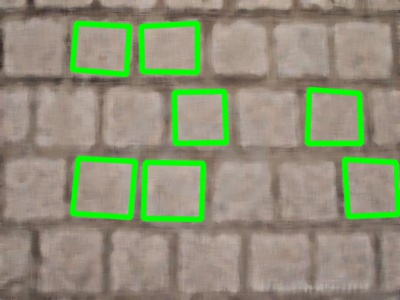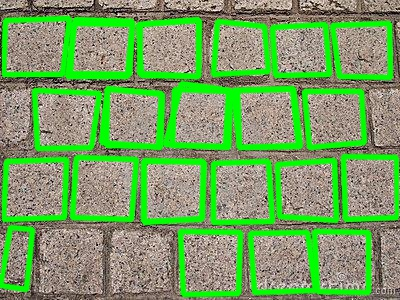# 6. 后记¶